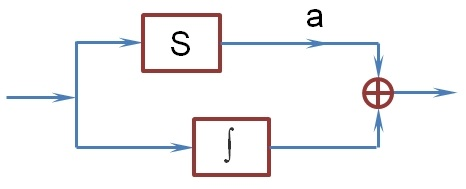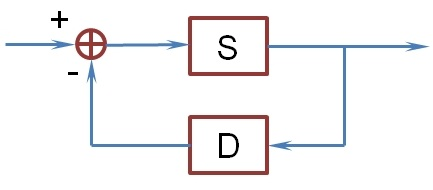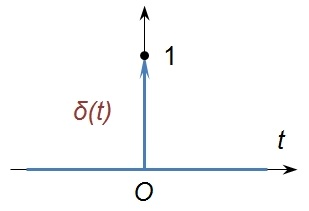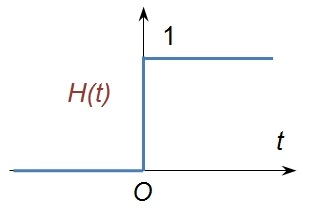# 1. 信号与系统

## 1.1 序言

实话说，我之前对信号系统一窍不通，但因为工作需要不得不学习它的基础知识。快餐式的学习又不是我的性格，于是就上手了奥本海姆的两本大部头经典，经过一番死磕，总算是把基本内容梳理完了。由于完全没有实战经验，我对信号系统的理解还很浅薄，加上所需数学知识还没有准备完善，学习过程中总是心里没底。但整理过程中，我仍然试图去了解问题的本质，即便有很多猜测和自以为的成分，却已是尽量做到逻辑完整、自洽。

信号学的内容比较多，以它为基础的延伸学科已然在各领域开枝散叶，比如语音信号处理和图形信号处理，都是如今AI某些分支的理论基础。万丈高楼平地起，本系列博客只打算学习经典信号学的基本概念和结论，更高阶的信号处理方法将放到《现代信号处理》专题里讨论。鉴于本人理论先行的习惯，打算还是先对连续信号建立较完整的理论，后面再集中讨论离散信号的不同、以及具体的处理策略，与奥本海姆的并行策略不同。

信号系统虽是一门应用学科，但就其理论体系而言，却是独立于具体的技术场景之外的。所以这里我也将不拿具体场景为例，而是把它当成一门纯粹的应用数学和算法设计课程来看待，注意力仍然集中在对讨论对象的定义、性质方法的推导、直至计算机的算法实现。教材虽厚，但大部分都是例题和习题，其实真正的理论部分内容有限。建议先粗略了解梗概，再细致推演细节，有数学基础的朋友甚至可以尝试自己“顺水推舟”，可能比直接看书更快、更深刻。

## 1.2 信号

课程名称就已经把它研究的对象概况清楚了：“信号”和“系统”。但我们肯定没法讨论任意的信号和系统，而只能针对某些广泛存在的、具有明确特征的信号与系统。具体而言，这里讨论的信号都可以抽象为一个实数域上的函数$x(t)$。由于大部分信号都体现出时序性，故这里的自变量也就用代表时间的$t$表示，但在讨论时还是仅作为一般的实（复）函数看待。

既然是一般实数域函数，那些我们已经熟知的函数性质和变换，这里就不赘述了。只是在信号系统中，它们有着更应景的名称，比如式（1）中的信号分别称为对信号$x(t)$的时移时间反转时间尺度变换。而更一般地，信号$x(\alpha t+\beta)$是对原信号时间轴的线性变换，想必你已经清楚它跟原信号之间的关系。

$x(t-t_0);\;\;x(-t);\;\;x(kt)\tag{1}$

还有类似偶信号奇信号周期信号的定义也都是简单直观的，其中周期信号满足$x(t)=x(t+T)$，它时移$T$后能与原信号重合。不难看出$kT$（$k$为整数）都是它的周期，而函数最小的周期被称为基波周期。但要注意，并不是所有的函数都有最小周期，比如常数函数、比如狄利克雷函数（有理数值为1、无理数处值为0）。教材里说除了常数函数都有基波周期，个人认为有误。

## 1.3 系统

“系统”被视为一个过程，它以一些信号作为输入，并以一定特征输出另一些信号。这样的描述和函数的定义并无本质差别，所以一个信号系统其实是一个广义的函数，只不过它的自变量和因变量都是实变量函数。相当一部分系统其实只有一个输入输出，故今后提到的系统都是指单输入输出系统$x(t)\to y(t)$。另外，简单的小系统可以通过综合运算，组合成更大的系统，这样的分拆思想是研究复杂系统的关键方法。

方框图可以为复杂系统提供直观的表示，图中的子系统一般用方框表示，像常用的积分、微分一般直接用$\int,D$表示。更简单的乘常数系统，可以直接在箭头上标出系数$a$。加法器$\bigoplus$是方框图中的常用部件，它一般用作多个系统的并联。相应地，复合系统$S2(S1(x(t)))$称为系统的串联级联。右图中由两个子系统和一个加法器组成的系统也很常见（D系统的输出一般为负因子），它被称为反馈互联确定系统特性，是分析或设计系统之前必须做的事情。而且针对普遍特性的研究，才更容易有理论结果和实用价值，这里先介绍几个常见的系统特性。首先对一般的有界函数$x(t)$，总希望系统的输出$y(t)$也是有界的，这样的系统称为稳定的。以时间为自变量的系统中，往往$y(t_0)$的值与$x(t)$的$t_0$时刻之后的值无关，这样的系统叫因果系统。如果$y(t_0)$仅仅与$x(t_0)$有关，它也叫无记忆系统。以上几个系统性质比较符合经验特性，但一定程度上限制了系统的处理范围，对更广义的系统不一定成立，故今后不作为默认性质。真正对系统分析有用的，其实是下面要介绍的两个性质。

对输入信号最简单的变换，无非就是时移和叠加，很多重要系统对这些变换仍然有“不变”的输出。首先我们希望系统的输出不受输入信号时移的影响，即如果$x(t)\to y(t)$，那么$x(t-t_0)\to y(t-t_0)$。输出函数随输入函数一起时移，这样的系统称为时不变的。线性组合是信号的最简单复合，如果系统还能满足式（2），它就被称为线性系统。线性时不变系统普遍存在，而且它还有着非常完善的理论成果，因此本系列把线性时不变系统（LIT）作为默认系统，并透彻讨论它的特性。但要注意：在对陌生系统做分析时，一定要先确认它是LIT系统。

$x_1(t)\to y_1(t);\;x_2(t)\to y_2(t)\;\;\Rightarrow\;\;ax_1(t)+bx_2(t)\to ay_1(t)+by_2(t)\tag{2}$

# 2. LIT系统

## 2.1 单位冲激函数

为了得到LIT系统的一般表征，还是要结合“时不变”和“线性”两大特性，对任意输入$x(t)$作一个线性拆解。设$\Delta(t-t_0)$是仅在$t_0$处有非零值$1$的函数，则$x(t)$就是所有函数$x(t_0)\Delta(t-t_0)$的叠加（$t_0$取遍所有实数）。进而如果$\Delta(t)$的响应函数为$Y(t)$，那么$x(t)$的响应函数$y(t)$便是所有函数$x(t_0)Y(t-t_0)$的叠加。

由于$t_0$取遍所有实数，“累加”的确切意义便是积分，以下先把$t_0$视为连续变量并重新记为$\tau$。现在需要构造一个等价于$\Delta(t-\tau)$的微分$\delta(t-\tau)\,\text{d}\tau$，也就是说微分仅在$\tau=t$处有非零值$1$，那就要求$\delta(t-\tau)$在$t$处有无穷大值且积分（面积）为$1$。为此需要定义一个满足式（3）的特殊的函数$\delta(t)$，它被称为单位冲激函数，坐标系中往往以一个单位长度的向上向量表示（左图）。在此定义下，$x(t)$便有了如式（4）积分表达式。$\delta(t)=\left\{\begin{matrix}\infty,&(t=0)\\0,&(t\ne 0)\end{matrix}\right.,\;\;\int_{-\infty}^{\infty}\delta(t)\,\text{d}t=1\tag{3}$

$x(t)=\int_{-\infty}^{\infty}x(\tau)\delta(t-\tau)\,\text{d}\tau\tag{4}$

教材上一般会用函数列的极限来解释$\delta$函数，但我们心里明白，式（3）的定义有严重的逻辑缺陷，一个值为无穷大的函数根本不能算作函数。这样的问题在工科教材上是无法解释清楚的，因为透彻地理解$\delta(t)$还需要《实变函数》和《泛函分析》的知识。在那里它叫狄拉克$\delta$函数，它是一个线性泛函，具体说是满足$\delta(\text{d}t)=\text{d}H(t)$的一个测度，其中$H(t)$是右图的Heviside阶梯函数

$\delta(t)$的严格定义是满足式（4）的泛函，注意这里的函数已经不是一般意义的函数，甚至积分（勒贝格积分）也不是一般意义的积分（黎曼积分）。另外，还可以把$\delta(t)$看成一个广义的分布函数，这其实是对本课程非常适合的角度。相比一般函数，它们被称为奇异函数，数学上已经严格证明，奇异函数和常规函数之间可以正常进行四则运算以及微积分，而且计算的结果与直观的理解是一致的，以后可以放心使用。

## 2.2 LIT的分解

有了式（4）对输入信号$x(t)$分解，就可以继续讨论系统的输出。在微分意义下，把响应$Y(t-\tau)$写作$h(t-\tau)\,\text{d}\tau$，那么响应函数$y(t)$就是式（5），它就是LIT系统的表征函数。虽然一般把$h(t)$称为单位冲激响应，但形式$h(t-\tau)\,\text{d}\tau$才是真正意义上的响应函数。就好比把$\delta(t-\tau)\,\text{d}\tau$视为真正的输入信号，而$\delta(t-\tau),\,h(t-\tau)$只是响应系数而已。理清这个关系，在今后的推导中才不会困惑。

$x(t)\,\to\, y(t)=\int_{-\infty}^{\infty}x(\tau)h(t-\tau)\text{d}\tau\tag{5}$

刚才一直是把式（5）看成函数的叠加，如果在某个固定的时间点$t=t_0$观察这个式子，$h(t_0-\tau)$显然表示时间点$\tau$的单位冲激在$t_0$时刻的响应系数，而$y(t_0)$就是所有时间的响应叠加。在这样的理解下，我们就比较容易讨论因果性系统的输出函数。因果系统每个时刻的信号只能对之后的输出造成影响，在函数上的表现就是：当$\tau>t_0$时$h(t_0-\tau)=0$（也可以说，当$t_0<0$时$h(t_0)=0$）。使用换元法整理式（5），便得到因果LIT系统的表征函数如式（6）所示。特别地，无记忆系统的单位冲激响应可以计作$h(t)=k\delta(t)$，系统表征函数简化为$y(t)=kx(t)$，这就是我们直觉上认为“应该是”的那个线性系统。

$x(t)\,\to\, y(t)=\int_0^{\infty}x(t-\tau)h(\tau)\text{d}\tau\tag{6}$

对于一个稳定系统，要求$x(t)$有限时$y(t)$也有限，用反证法可知$h(t)$是绝对可积的（式（7））。反之如果式（7）成立，也不难证明$y(t)$有界（取$|x(t)|$的上限），从而我们知道：单位冲激响应绝对可积是系统稳定的充要条件。稳定系统的$h(t)$一般表现为渐进趋于$0$，如果它在有限时间后只有$0$值，这样的响应也叫有限冲激响应FIR），这种系统也比较常见。另外，$h(t)$收敛于0的速度，一定程度上体现了系统响应的快慢。

$\int_{-\infty}^{+\infty}|h(\tau)|\,\text{d}\tau<\infty\tag{7}$

## 2.3 卷积

LIT的表征函数（5）由两个函数生成另一个函数，这样函数间的“运算”在实变函数里叫算子，而式（5）这样的算子被称为卷积。卷积就好像是把其中一个函数反转，然后移动着和另一个函数积分，明白式（5）的物理意义后，也能类推这种算子的作用了。一般把函数的卷积计作$x(t)*y(t)$，由式（5）中$x(t),h(t)$角色的对称性，不难得知卷积满足交换律（式（8））。

$x(t)*y(t)=y(t)*x(t)\tag{8}$

另外也不难证明（实变函数课程中有详细证明，也并不难），卷积还满足式（9）（10）的分配率结合律（结合律本质上跟矩阵乘法的结合律相同）。既然LIT的表征函数是一个卷积$x(t)*h(t)$，再根据卷积的运算律，就能得到LIT系统的一些主要性质。比如系统$h_1(t),h_2(t)$并联后的系统满足：单位冲激响应为$h_1(t)+h_2(t)$；而串联（级联）后的系统满足：单位冲激响应为$h_1(t)*h_2(t)$，且串联的子系统可以任意调换顺序。反之如果系统的单位冲激响应可拆解为多个简单的响应，则可以方便地将系统拆解为子系统。

$x(t)*[y(t)+z(t)]=x(t)*y(t)+x(t)*z(t)\tag{9}$

$x(t)*[y(t)*z(t)]=[x(t)*y(t)]*z(t)\tag{10}$

不难验证，微分、积分本身就是LIT系统，根据卷积的结合律便有式（11）（12）成立，它们是卷积和微积分运算的互换法则。为了显式表示微积分的单位冲激响应，先考察恒定变换$x(t)\to x(t)$，根据式（4）可知它的单位冲激响应就是$\delta(t)$。利用结合律可知，微、积分系统的单位冲激响应就是$\delta(t)$的微、积分。把$\delta(t)$计作$u_0(t)$，它的（高阶）微积分计作$u_n(t)$（式（13），$n\leqslant 0$时都是奇异函数）。其中$u_{-1}(t)$就是上面提到的Heviside阶梯函数，它也被称为阶跃响应函数，简计作$u(t)$。再一次使用结合律，容易有式（14）的性质。

$\dfrac{\text{d}}{\text{d}t}[x(t)*y(t)]=x(t)*\dfrac{\text{d}y(t)}{\text{d}t}\tag{11}$

$\int_{-\infty}^tx(\tau)*y(\tau)\,\text{d}\tau=x(t)*\int_{-\infty}^ty(\tau)\,\text{d}\tau\tag{12}$

$u_{n}(t)=\dfrac{\text{d}^n\delta(t)}{\text{d}t^n};\;\;u_{-n}(t)=\int_{-\infty}^t\dots\int_{-\infty}^\tau\delta(\tau)\,\text{d}\tau\tag{13}$

$u_m(t)*u_n(t)=u_{m+n}(t),\;\;(m,n\in\Bbb{Z})\tag{14}$

博客总目录这里

【前序学科】微积分、傅里叶分析、常微分方程

【参考资料】

  《信号与系统（2nd）》，奥本海姆，2013

本课程的主要参考书，内容详实，有丰富的例题、习题、以及现实中具体的问题。数学部分适合起点低的同学，但个人感觉缺少对本质的分析。过于周全的缺点便是，难以突显核心的结论，以及不能将关键信息立体地串联起来。

 《信号与系统（3nd）》，郑君里，2011

大概是国内最好的参考书了，编排和讲法更符合国人的阅读习惯。对数学的论证没有刻意回避，虽然偶有瑕疵，总体比奥本的书有更多信息量。

 《信号与系统》，陈后金，2007

以简洁实用为编排目的，内容干净凝练，适合快速上手。

posted on 2020-02-07 10:00  卞爱华  阅读(677)  评论(0编辑  收藏